You may find a pencil and paper handy for this quiz.

# Algebra - Order of Operations Revisited

This Math quiz is called 'Algebra - Order of Operations Revisited' and it has been written by teachers to help you if you are studying the subject at middle school. Playing educational quizzes is a fabulous way to learn if you are in the 6th, 7th or 8th grade - aged 11 to 14.

It costs only \$12.50 per month to play this quiz and over 3,500 others that help you with your school work. You can subscribe on the page at Join Us

Can you imagine what it would be like if math was done differently from one country to another? At the very least, it would cause economic chaos. So, although the world is divided into multiple different cultures and languages, mathematicians throughout the world worked together over the centuries to develop a common formula and method to solving all mathematical problems.

Addition was easy enough as was subtraction. Multiplication and division even began as a universal understanding. However, what if you had mathematical problems that included addition, subtraction, multiplication, division, positive and negative numbers and exponents all in one problem? How would you go about solving that problem?

In order to solve the problem for the world, an Order of Operations was created and approved and accepted by all countries. You more than likely are already quite familiar with the Order of Operations as you learned it in your earlier grades but it might have been a couple of years since you put any focus on it or applied it to more complex problems. As math is becoming more detailed, involving more equations, it is important to revisit and work with the Order of Operations so that you know how to find the correct solution in a mixed mathematical problem. With that focus, this quiz has been set up to see what you remember about working with the Order of Operation.

Here is a little reminder or hint on the Order:

P = Parentheses
E = Exponents (Powers and Square Roots)
MD = Multiplication and Division (Left to Right)
AS = Addition and Subtraction (Left to Right)

The problems contained within parentheses are always worked out first.

If a number has an exponent, then we would raise that number next. For example, if you had 53 you would work out the problem as 5 x 5 = 25 x 5 = 125. The 125 would replace the number that held the exponent so 53 would now be rewritten as 125.

Next you do multiplying and division from left to right. This does not mean you multiply first and then divide but, rather that you work from left to right so if the first problem was a multiplication then you multiply first and if it is a division problem then you would divide first. For example, let’s look at the following two simple problems.

24 ÷ 6 x 3 = (This problem has division first so you would first divide 24 ÷ 6 = 4 and then you would multiply 4 x 3 = 12 so that 24 ÷ 6 x 3 = 12. If you multiplied 6 x 3 = 18 first and then divided 24 ÷ 16 = 1.5, the answer would be wrong because you didn’t follow the Order of Operation.)

18 x 12 ÷ 3 = (This problem has multiplication first so you would first multiply 18 x 12 = 216 and then you would divide 216 ÷ 3 = 72 so that 18 x 12 ÷ 3 = 72. Interestingly enough with this particular problem, if you divide 12 ÷ 3 = 4 and then multiply 18 x 4 = 72, you get the same answer but you still need to follow the Order of Operation by moving left to right to be certain you get the right answer.)

Finally, the last stage of the Order of Operation is add and subtract left to right and just like multiplication and division, if the first numbers are added then you add, if they are subtraction, then you subtract. Always work left to right.

Now it’s time to see if you can correctly work the following ten problems using the Order of Operations to find the answer to the solution. If you are ready - then begin!

1.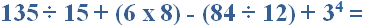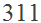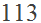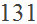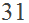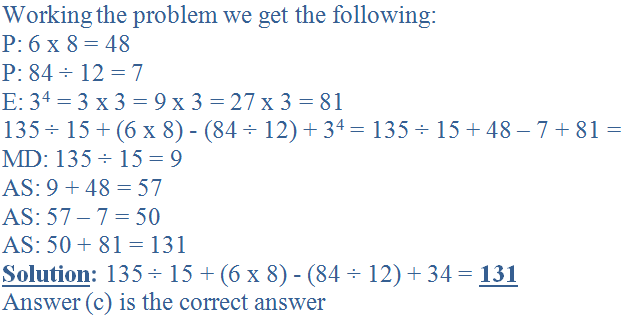2.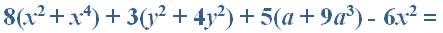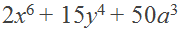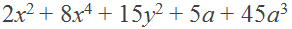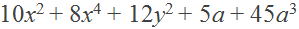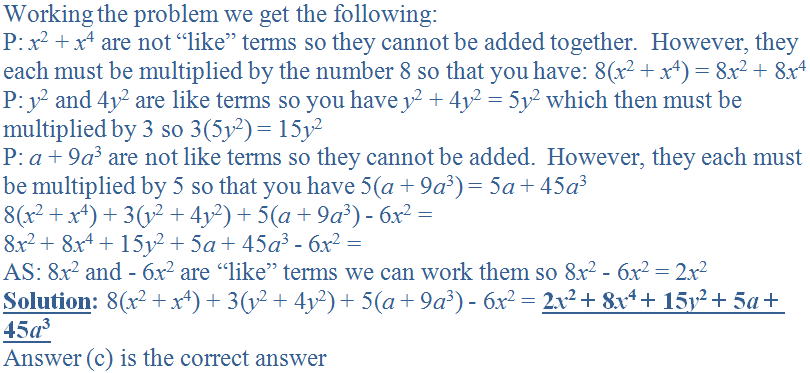3.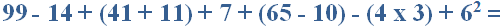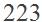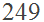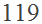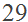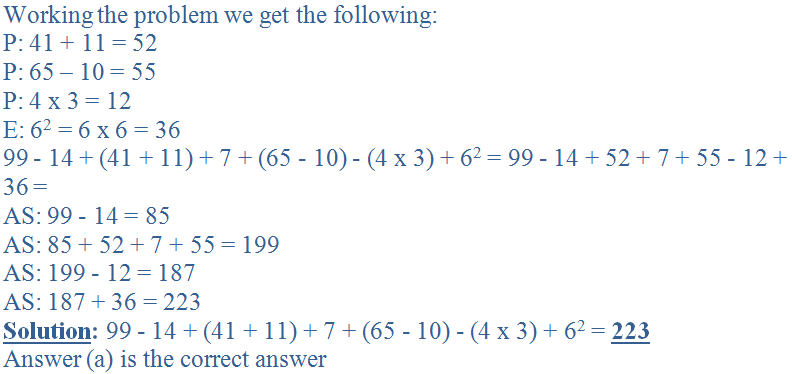4.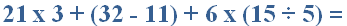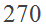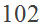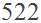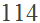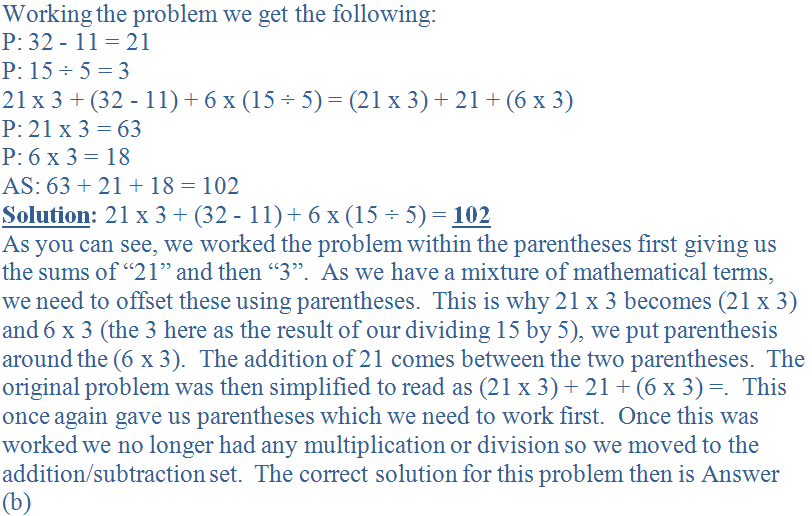5.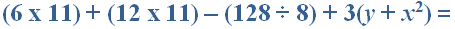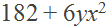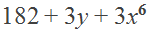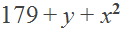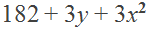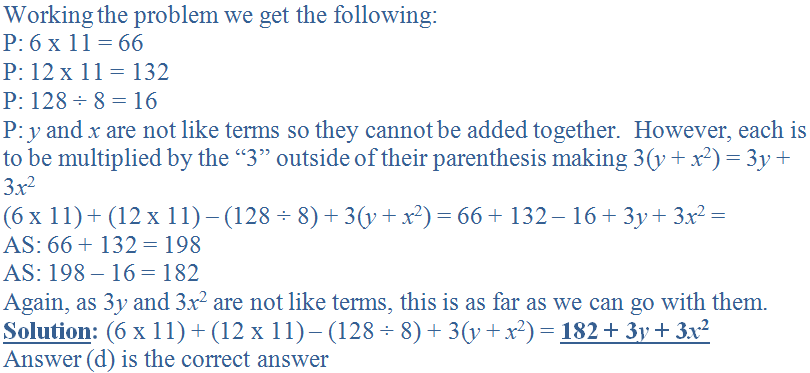6.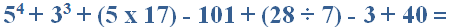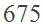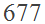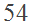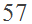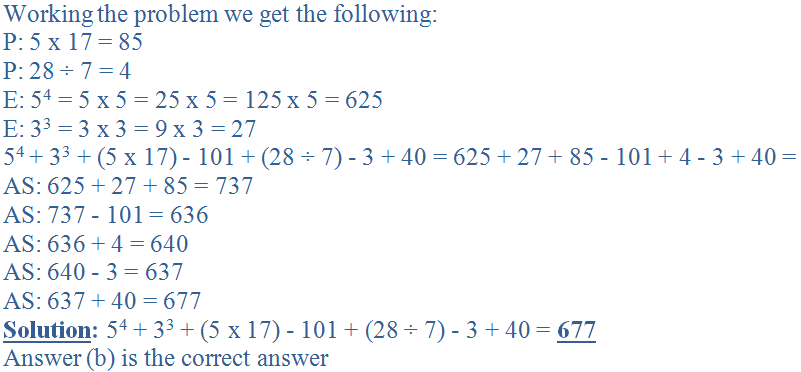7.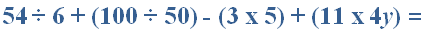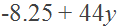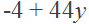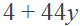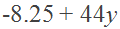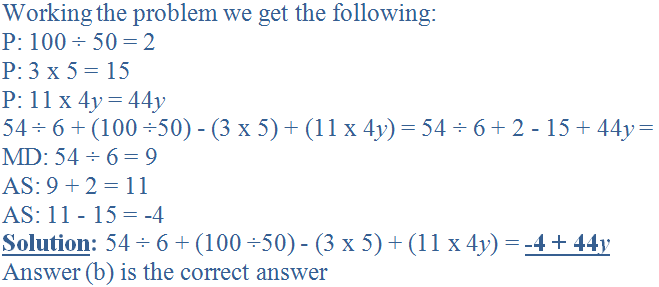8.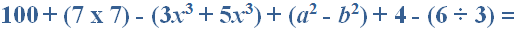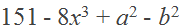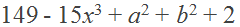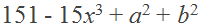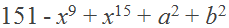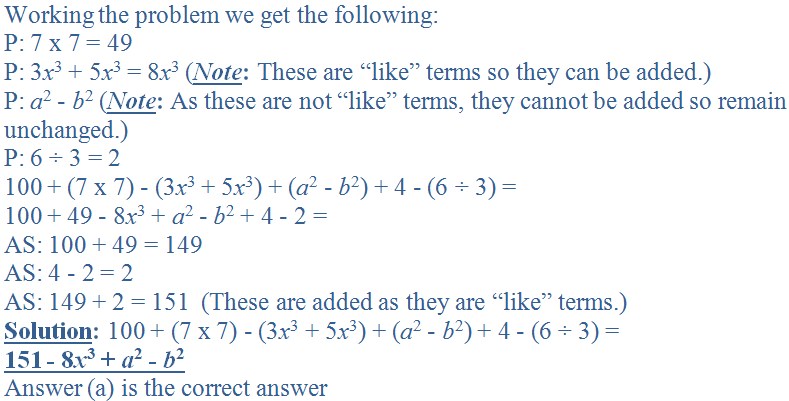9.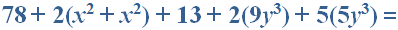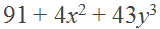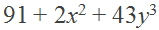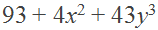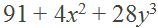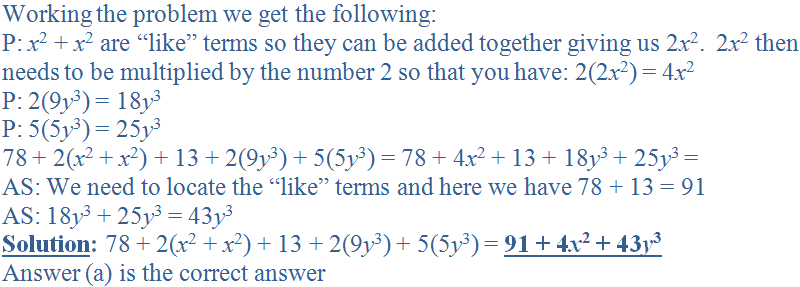10.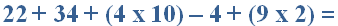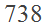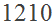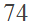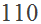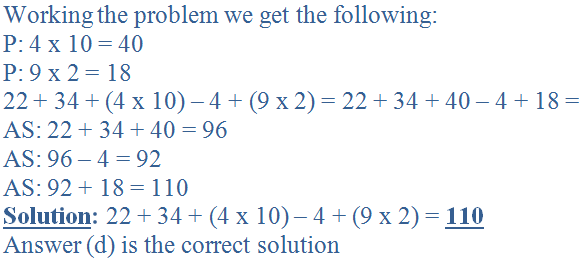Author:  Christine G. Broome